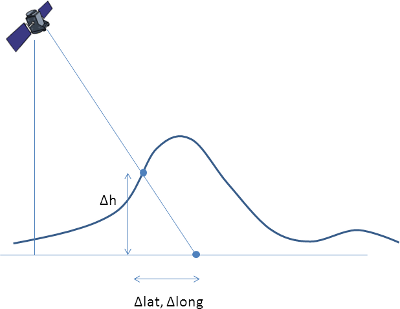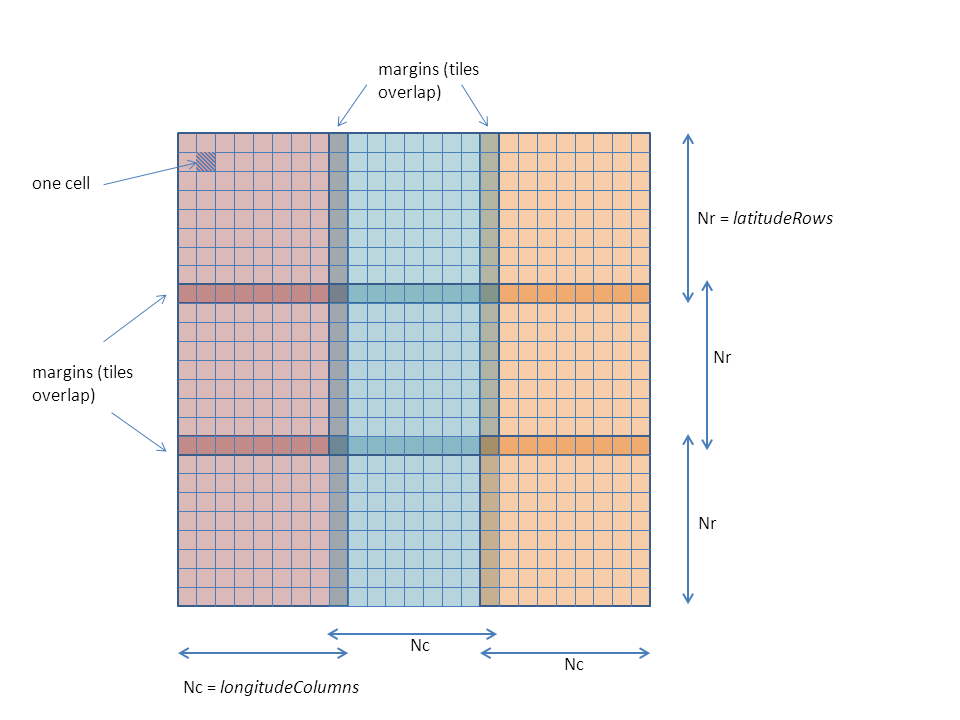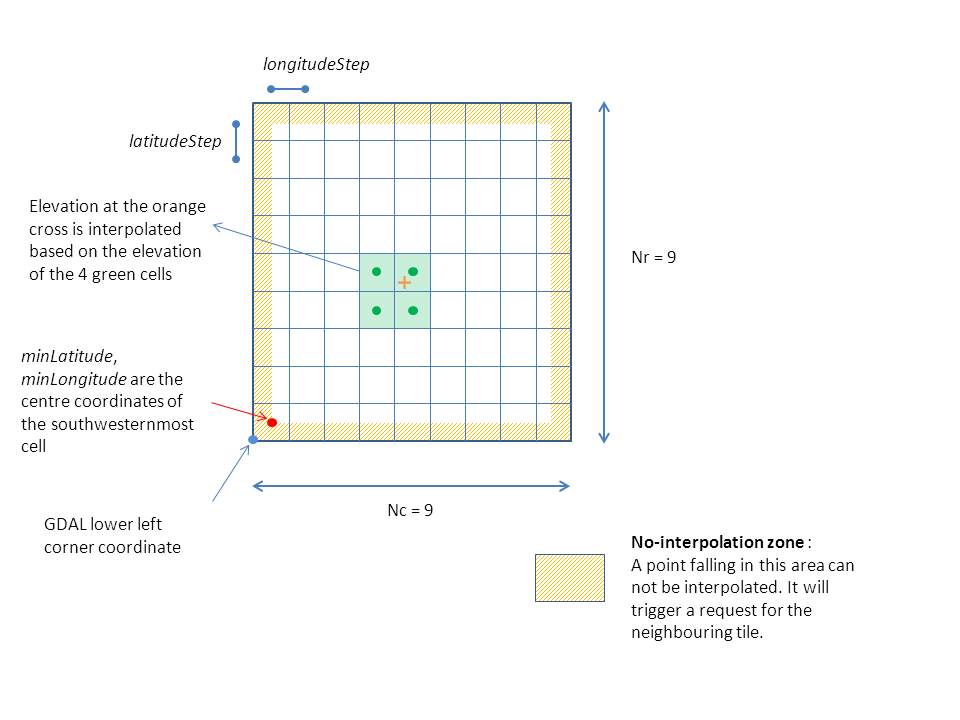Direct Location with a DEM

The aim of this tutorial is to compute a direct location grid by intersection of the line of sight with a DEM (Digital Elevation Model), using Duvenhage’s algorithm. This algorithm is the most performant one in Rugged.

The following figure shows the effects of taking into account the DEM in the computation of latitude, longitude and altitude:Feeding Rugged with DEM tiles

Rugged does not parse DEM files but takes buffers of elevation data as input. It is up to the calling application to read the DEM and load the data into buffers. Rugged provides a tile mecanism with cache for large DEMs allowing the user to load one tile at a time. This is in line with the format of world coverage DEMs such as SRTM. Rugged offers an interface for updating the DEM tiles in cache with a callback function triggered everytime a coordinate falls outside the current region.

The calling application must implement the callback function for loading the tiles. We recommend to use GDAL (http://www.gdal.org/) to parse the DEM files as it handles most formats (including geoTIFF for Aster DEM or DTED for SRTM). Rugged does not include the parsing of the DEM, by design, and yet we could have used GDAL. We want Rugged to remain a low-level library that does not pull too many third-party libraries.

In this tutorial, we will not include real DEM data. Instead we are going to create a fake DEM representing a volcano in a form of a perfect cone, similar to the Mayon volcano in the Philippines, except that we will locate it somewhere just below our satellite. This example is already part of Rugged tests cases, the source code is available in the package org.orekit.rugged.raster, file VolcanicConeElevationUpdater.java.

The class VolcanicConeElevationUpdater implements the interface TileUpdater with its method updateTile. The method is in charge of loading a tile. The extent of the tile must be such that it covers at least the ground point with coordinates (latitude, longitude) which are passed as arguments to the method. The tile is an object of type UpdatableTile which has two methods :

• setGeometry(minLatitude, minLongitude, latitudeStep, longitudeStep, latitudeRows, longitudeRows) : initializes the extent of the new tile before loading

• setElevation(latIdx, longIdx, elevation) : fills the tile buffer at indices (latIdx, lonIdx) with value elevation

Here’s the source code of the class VolcanicConeElevationUpdater :

```import org.hipparchus.util.FastMath;
import org.orekit.rugged.raster.TileUpdater;
import org.orekit.rugged.raster.UpdatableTile;
import org.orekit.bodies.GeodeticPoint;
import org.orekit.rugged.errors.RuggedException;
import org.orekit.utils.Constants;

public class VolcanicConeElevationUpdater implements TileUpdater {

private GeodeticPoint summit;
private double        slope;
private double        base;
private double        size;
private int           n;

public VolcanicConeElevationUpdater(GeodeticPoint summit, double slope, double base,
double size, int n) {
this.summit = summit;
this.slope  = slope;
this.base   = base;
this.size   = size;
this.n      = n;
}

public void updateTile(double latitude, double longitude, UpdatableTile tile)
throws RuggedException {
double step         = size / (n - 1);
double minLatitude  = size * FastMath.floor(latitude  / size);
double minLongitude = size * FastMath.floor(longitude / size);
double sinSlope     = FastMath.sin(slope);
tile.setGeometry(minLatitude, minLongitude, step, step, n, n);
for (int i = 0; i < n; ++i) {
double cellLatitude = minLatitude + i * step;
for (int j = 0; j < n; ++j) {
double cellLongitude = minLongitude + j * step;
FastMath.hypot(cellLatitude  - summit.getLatitude(),
cellLongitude - summit.getLongitude());
double altitude = FastMath.max(summit.getAltitude() - distance * sinSlope,
base);
tile.setElevation(i, j, altitude);
}
}
}

}
```

Important notes on DEM tiles :

• Ground point elevation are obtained by bilinear interpolation between 4 neighbouring cells. There is no specific algorithm for border management. As a consequence, a point falling on the border of the tile is considered outside. DEM tiles must be overlapping by at least one line/column in all directions.

• In Rugged terminology, the minimum latitude and longitude correspond to the centre of the farthest Southwest cell of the DEM. Be careful if using GDAL to pass the correct information as there is half a pixel shift with respect to the lower left corner coordinates in gdalinfo.

The following diagram illustrates proper DEM tiling with one line/column overlaps between neighbouring tiles :This diagram tries to represent the meaning of the different parameters in the definition of a tile :Initializing Rugged with a DEM

The initialization step differs slightly from the first tutorial Direct location, as we need to pass the information about our TileUpdater.

Instantiate an object derived from TileUpdater :

```TileUpdater updater = new VolcanicConeElevationUpdater(summit, slope, base, FastMath.toRadians(1.0), 100);

int nbTiles = 8 ; //number max of tiles in Rugged cache
AlgorithmId algoId = AlgorithmId.DUVENHAGE;
```

Initialize Rugged with these parameters :

```Rugged rugged = new RuggedBuilder().
setDigitalElevationModel(updater, nbTiles).
setAlgorithm(algoId).
setEllipsoid(EllipsoidId.WGS84, BodyRotatingFrameId.ITRF).
setTimeSpan(startDate, stopDate, 0.1, 10.0).
setTrajectory(InertialFrameId.EME2000,
satellitePVList, 6, CartesianDerivativesFilter.USE_P,
satelliteQList, 8, AngularDerivativesFilter.USE_R).
build();
```

Computing a direct location grid

In a similar way as in the first tutorial Direct Location, we call Rugged direct location method. This time it is called in a loop so as to generate a full grid on disk.

```DataOutputStream dos = new DataOutputStream(new FileOutputStream("demDirectLoc.c1"));
int lineStep = (maxLine - minLine) / nbLineStep;
int pxStep = (maxPx - minPx) / nbPxStep;

for (int i = 0; i < nbLineStep; i++) {

List<GeodeticPoint> pointList = new ArrayList<GeodeticPoint>(nbPxStep);
int currentLine = minLine + i * lineStep;
for (int j = 0; j < nbPxStep; j++) {
int currentPx = minPx + j * pxStep;
// Call to direct localization on current line
Vector3D position = lineSensor.getPosition();
AbsoluteDate currentLineDate = lineSensor.getDate(currentLine);
Vector3D los = lineSensor.getLOS(absDate, currentPx);
}
for (GeodeticPoint point : pointList) {
if (point != null) {
dos.writeFloat((float) FastMath.toDegrees(point.getLatitude()));
} else {
dos.writeFloat((float) base);
}
}
for (GeodeticPoint point : pointList) {
if (point != null) {
dos.writeFloat((float) FastMath.toDegrees(point.getLongitude()));
} else {
dos.writeFloat((float) base);
}
}
for (GeodeticPoint point : pointList) {
if (point != null) {
dos.writeFloat((float) point.getAltitude());
} else {
dos.writeFloat((float) base);
}
}
}
```

Source code

The source code is available in DirectLocationWithDEM.java (package fr.cs.examples under src/tutorials)

Top of the page